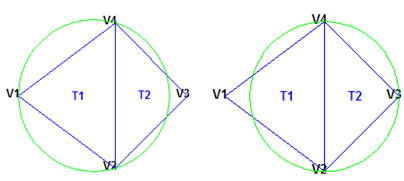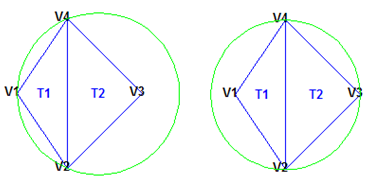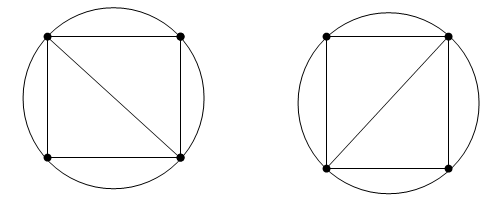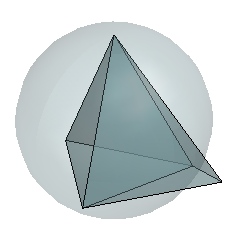## 使用 Delaunay 三角剖分

### Delaunay 三角剖分的定义

Delaunay 三角剖分广泛应用于许多不同应用程序中的科学计算。虽然有大量的计算三角剖分的算法，但 Delaunay 三角剖分以其实用的几何属性广受欢迎。Delaunay 三角剖分堪称“外形整齐”，原因在于为满足空外接圆属性，优先选择带有较大内角的三角形，而不是带有较小内角的三角形。非 Delaunay 三角剖分中的三角形在顶点 `V2``V4` 处呈锐角。如果将 `{V2, V4}` 边替换为连接 `V1``V3` 的边，会实现最小角的最大化并且使得该三角剖分变为 Delaunay 三角剖分。另外，Delaunay 三角剖分将最近邻点的点连接在一起。这两个特征（外形整齐和最近邻点关系）在实践中具有重要的作用，有助于促进在散点数据插值中使用 Delaunay 三角剖分。Delaunay 三角剖分的属性扩展到更高的维度。三维点集的三角剖分由四面体组成。下图显示了一个简单的由 2 个四面体组成的三维 Delaunay 三角剖分。显示一个四面体的外接球以突出显示空外接球规则。### 创建 Delaunay 三角剖分

MATLAB® 提供两种创建 Delaunay 三角剖分的方法：

`delaunay` 函数支持创建二维和三维 Delaunay 三角剖分。`delaunayn` 函数支持创建四维或更高维度的 Delaunay 三角剖分。

`delaunayTriangulation` 类支持创建二维和三维 Delaunay 三角剖分。它提供了多种适用于开发基于三角剖分的算法的方法。这些类方法与函数相似，但它们仅限于处理使用 `delaunayTriangulation` 创建的三角剖分。`delaunayTriangulation` 类还支持创建相关构造，例如凸包和 Voronoi 图。它还支持创建约束性 Delaunay 三角剖分。

• 在仅需要基本三角剖分数据并且数据对应用程序已足够完整时，`delaunay` 函数非常有用。

• `delaunayTriangulation` 类提供了更多功能用于开发基于三角剖分的应用程序。在需要三角剖分并且希望执行以下任意操作时，该类非常有用：

• 在三角剖分中搜索包含查询点的三角形或四面体。

• 使用三角剖分执行最近邻点搜索。

• 查询三角剖分的拓扑邻接或几何属性。

• 修改三角剖分以插入或删除点。

• 约束三角剖分中的边界 - 这称为约束性的 Delaunay 三角剖分。

• 对多边形进行三角剖分并（可选）删除域外部的三角形。

• 使用 Delaunay 三角剖分计算凸包或 Voronoi 图。

#### 使用 delaunay 和 delaunayn 函数

`delaunay``delaunayn` 函数获取点集并生成矩阵格式的三角剖分。请参阅三角剖分矩阵格式以了解有关该数据结构体的详细信息。在二维模式下，`delaunay` 函数通常用于生成此类三角剖分，即它可用于绘制根据一组分散数据点来定义的曲面。在该应用中，必须注意该方法仅在曲面为单值型时可用。例如，它不能用于绘制球面，因为有两个 `z` 值对应于同一个 (`x`, `y`) 坐标。通过一个简单示例来演示 `delaunay` 函数如何可用于绘制表示抽样数据集的曲面。

```load seamount plot(x,y,'.','markersize',12) xlabel('Longitude'), ylabel('Latitude') grid on``````tri = delaunay(x,y); hold on, triplot(tri,x,y), hold off``````figure hidden on trimesh(tri,x,y,z) xlabel('Longitude'),ylabel('Latitude'),zlabel('Depth in Feet');``````rng('default') X = rand([30 3]); tet = delaunay(X); faceColor = [0.6875 0.8750 0.8984]; tetramesh(tet,X,'FaceColor', faceColor,'FaceAlpha',0.3);```MATLAB 提供 `delaunayn` 函数以支持创建四维及更高维的 Delaunay 三角剖分。此外，还提供了两个补充函数 `tsearchn``dsearchn`，以支持对 N 维三角剖分执行空间搜索。有关基于三角剖分的搜索的详细信息，请参阅空间搜索

#### 使用 delaunayTriangulation 类

`delaunayTriangulation` 类提供另一种在 MATLAB 中创建 Delaunay 三角剖分的方法。虽然 `delaunay``delaunayTriangulation` 使用相同的基本算法并生成相同的三角剖分，但 `delaunayTriangulation` 提供了适用于开发基于 Delaunay 的算法的补充方法。这些方法与函数类似，可以将它们与三角剖分数据一起打包到称为类的容器中。将所有内容集中在类中，这样可以提供更加有条理的结构，从而提高易用性。它还可以提高基于三角剖分的搜索的性能，例如点位置和最近邻位置。`delaunayTriangulation` 支持对 Delaunay 三角剖分进行增量编辑。还可以实行二维中的边约束。

```load seamount DT = delaunayTriangulation(x,y)```
```DT = delaunayTriangulation with properties: Points: [294x2 double] ConnectivityList: [566x3 double] Constraints: [] ```

`delaunayTriangulation` 类是矩阵数据的包装器，并且提供了一组补充方法。可以按照访问结构体字段的方式访问 `delaunayTriangulation` 中的属性。

`DT.Points;`

`DT.ConnectivityList;`

`DT.ConnectivityList(1,:)`
```ans = 1×3 230 205 152 ```

`delaunayTriangulation` 提供了一种对 `ConnectivityList` 属性矩阵进行索引的简单方法。

`DT(1,:)`
```ans = 1×3 230 205 152 ```

`DT(1,1)`
```ans = 230 ```

`DT(:,:);`

`delaunayTriangulation` 输出 `DT` 进行索引，这与对 `delaunay` 的三角剖分数组输出进行索引类似。二者的区别是您可以对 `DT` 调用额外方法（例如 `nearestNeighbor``pointLocation`）。

```triplot(DT); axis equal xlabel('Longitude'), ylabel('Latitude') grid on``````hold on k = convexHull(DT); xHull = DT.Points(k,1); yHull = DT.Points(k,2); plot(xHull,yHull,'r','LineWidth',2); hold off``````figure plot(xHull,yHull,'r','LineWidth',2); axis equal xlabel('Longitude'),ylabel('Latitude') grid on % The convex hull topology duplicates the start and end vertex. % Remove the duplicate entry. k(end) = []; % Now remove the points on the convex hull. DT.Points(k,:) = []```
```DT = delaunayTriangulation with properties: Points: [274x2 double] ConnectivityList: [528x3 double] Constraints: [] ```
```% Plot the new triangulation. hold on triplot(DT); hold off````vertexId = nearestNeighbor(DT, 211.6, -48.15)`
```vertexId = 50 ```

`DT.Points(vertexId,:) = []`
```DT = delaunayTriangulation with properties: Points: [273x2 double] ConnectivityList: [525x3 double] Constraints: [] ```

```figure plot(xHull,yHull,'r','LineWidth',2); axis equal xlabel('Longitude'),ylabel('Latitude') grid on hold on triplot(DT); hold off``````Padditional = [210.9 -48.5; 211.6 -48.5; ... 211.6 -47.9; 210.9 -47.9]; DT.Points(end+(1:4),:) = Padditional```
```DT = delaunayTriangulation with properties: Points: [277x2 double] ConnectivityList: [548x3 double] Constraints: [] ```

`close all`

```figure plot(xHull,yHull,'r','LineWidth',2); axis equal xlabel('Longitude'),ylabel('Latitude') grid on hold on triplot(DT); hold off````DT.Points(274,:) = [211 -48.4];`

`close all`

```figure plot(xHull,yHull,'r','LineWidth',2); axis equal xlabel('Longitude'),ylabel('Latitude') grid on hold on triplot(DT); hold off``````attTris = vertexAttachments(DT,274); hold on triplot(DT(attTris{:},:),DT.Points(:,1),DT.Points(:,2),'g') hold off````delaunayTriangulation` 也可以用于对三维空间中的点进行三角剖分。生成的三角剖分由四面体构成。

```rng('default') P = rand(30,3); DT = delaunayTriangulation(P)```
```DT = delaunayTriangulation with properties: Points: [30x3 double] ConnectivityList: [102x4 double] Constraints: [] ```
```faceColor = [0.6875 0.8750 0.8984]; tetramesh(DT,'FaceColor', faceColor,'FaceAlpha',0.3);````tetramesh` 函数会同时绘制三角剖分的内面和外面。对于大型三维三角剖分，绘制内面可能导致不必要的资源使用。绘制边界可能更加适宜。您可以使用 `freeBoundary` 方法获取矩阵格式的边界三角剖分。然后将结果传递给 `trimesh``trisurf`

#### 受约束的 Delaunay 三角剖分

`delaunayTriangulation` 类允许您约束二维三角剖分中的边界。这意味着可以选择三角剖分中的一对点并约束用于连接这些点的边。可以将其作为“施加压力”绘制一对或多对点之间的边界。以下示例说明了边约束对三角剖分的影响。`P = [2 4; 6 1; 9 4; 6 7];`

```C = [1 3]; DT = delaunayTriangulation(P,C);```

```triplot(DT) % Label the vertices. hold on numvx = size(P,1); vxlabels = arrayfun(@(n) {sprintf('V%d', n)}, (1:numvx)'); Hpl = text(P(:,1)+0.2, P(:,2)+0.2, vxlabels, 'FontWeight', ... 'bold', 'HorizontalAlignment','center', 'BackgroundColor', ... 'none'); hold off % Use the incenters to find the positions for placing triangle labels on the plot. hold on IC = incenter(DT); numtri = size(DT,1); trilabels = arrayfun(@(P) {sprintf('T%d', P)}, (1:numtri)'); Htl = text(IC(:,1),IC(:,2),trilabels,'FontWeight','bold', ... 'HorizontalAlignment','center','Color','blue'); hold off % Plot the circumcircle associated with the triangle, T1. hold on [CC,r] = circumcenter(DT); theta = 0:pi/50:2*pi; xunit = r(1)*cos(theta) + CC(1,1); yunit = r(1)*sin(theta) + CC(1,2); plot(xunit,yunit,'g'); axis equal hold off``````figure() axis([-1 17 -1 6]); axis equal P = [0 0; 16 0; 16 2; 2 2; 2 3; 8 3; 8 5; 0 5]; patch(P(:,1),P(:,2),'-r','LineWidth',2,'FaceColor',... 'none','EdgeColor','r'); % Label the points. hold on numvx = size(P,1); vxlabels = arrayfun(@(n) {sprintf('P%d', n)}, (1:numvx)'); Hpl = text(P(:,1)+0.2, P(:,2)+0.2, vxlabels, 'FontWeight', ... 'bold', 'HorizontalAlignment','center', 'BackgroundColor', ... 'none'); hold off``````figure() subplot(2,1,1); axis([-1 17 -1 6]); axis equal P = [0 0; 16 0; 16 2; 2 2; 2 3; 8 3; 8 5; 0 5]; DT = delaunayTriangulation(P); triplot(DT) hold on; patch(P(:,1),P(:,2),'-r','LineWidth',2,'FaceColor',... 'none','EdgeColor','r'); hold off % Plot the standalone triangulation in a subplot. subplot(2,1,2); axis([-1 17 -1 6]); axis equal triplot(DT)````C = [1 2; 2 3; 3 4; 4 5; 5 6; 6 7; 7 8; 8 1];`

`DT = delaunayTriangulation(P,C);`

```figure('Color','white') subplot(2,1,1); axis([-1 17 -1 6]); axis equal triplot(DT) hold on; patch(P(:,1),P(:,2),'-r','LineWidth',2, ... 'FaceColor','none','EdgeColor','r'); hold off % Plot the standalone triangulation in a subplot. subplot(2,1,2); axis([-1 17 -1 6]); axis equal triplot(DT)``````% Plot the constrained edges in red. figure('Color','white') subplot(2,1,1); plot(P(C'),P(C'+size(P,1)),'-r','LineWidth', 2); axis([-1 17 -1 6]); % Compute the in/out status. IO = isInterior(DT); subplot(2,1,2); hold on; axis([-1 17 -1 6]); % Use triplot to plot the triangles that are inside. % Uses logical indexing and dt(i,j) shorthand % format to access the triangulation. triplot(DT(IO, :),DT.Points(:,1), DT.Points(:,2),'LineWidth', 2) hold off;```### 包含重复位置的点集的三角剖分

MATLAB 中的 Delaunay 算法可以基于特征点集构建三角剖分。如果传递到三角剖分函数或类的点不是特征点，会检测到重复位置并忽略重复点。这样生成的三角剖分不会参照原始输入中的一些点，即重复点。使用 `delaunay``delaunayn` 函数时，重复情况的存在可能没什么影响。但是，由于 `delaunayTriangulation` 类提供的许多查询以索引为基础，因此必须了解 `delaunayTriangulation` 对特征数据集执行三角剖分和处理。因此，基于唯一点集创建索引是惯例。这些数据通过 `delaunayTriangulation``Points` 属性维护。

```rng('default') P = rand([25 2]); P(18,:) = P(8,:) P(16,:) = P(6,:) P(12,:) = P(2,:) DT = delaunayTriangulation(P) ```

```DT = delaunayTriangulation with properties: Points: [22x2 double] ConnectivityList: [31x3 double] Constraints: []```

```K = DT.convexHull(); plot(DT.Points(:,1),DT.Points(:,2),'.'); hold on plot(DT.Points(K,1),DT.Points(K,2),'-r');```

```K = DT.convexHull(); plot(P(:,1),P(:,2),'.'); hold on plot(P(K,1),P(K,2),'-r');```

```rng('default') P = rand([25 2]); P(18,:) = P(8,:) P(16,:) = P(6,:) P(12,:) = P(2,:) [~, I, ~] = unique(P,'first','rows'); I = sort(I); P = P(I,:); DT = delaunayTriangulation(P) % The point set is unique```